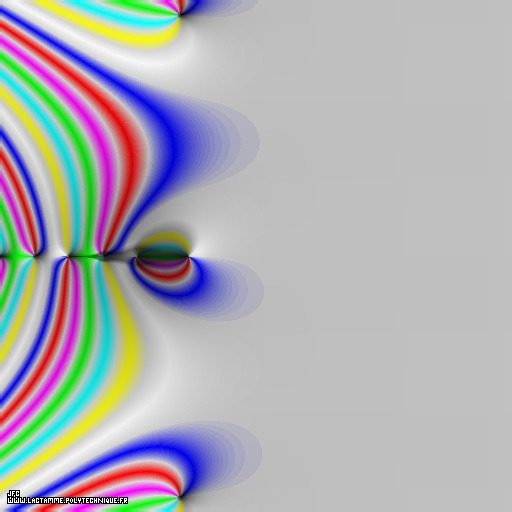Tridimensional display of the Riemann Zeta function inside [-10.0,+20.0]x[-15.0,+15.0] (bird's-eye view) [Visualisation tridimensionnelle de la fonction Zêta de Riemann dans [-10.0,+20.0]x[-15.0,+15.0] (vue aérienne)].

The real Zeta function is defined as the serie:
```                            n=+infinity
_______
\
\       -s
Zeta(s) =  /      n
/______

n=1

\-/ s > 1
```

The complex Riemann Zeta function is defined as the serie:
```                            n=+infinity
_______
\
\       -z
Zeta(z) =  /      n
/______

n=1

\-/ z : Re(z) > 1
```

or again (Leonhard Euler):
```                              _________
|     |
|     |      1
Zeta(z) =  |     |  ---------
|     |        -z
|     |   1 - p

p E P
```
where 'P' denotes the set of the prime numbers 'p'.

It can be computed for all z with the following analytic continuation:
```                               n=N-1
_______
\
\       -z
Zeta(z) =  /      n
/______

n=1

1-z      -z
N        N
+ ------ + -----
z-1       2

k=V                        p=2k-2
_______                     ________
\          B                 |    |
\          2k    -z-(2k)+1  |    |
+  /      [-------.N           |    | (z+p)]
/______   (2k)!              |    |

k=1                          p=0

+ epsilon(z,N,V)

\-/ z : Re(z+2V+1) > 1

N ~ |z|
```

This picture displays the modulus of the Riemann Zeta function as a surface in a tridimensional space (the two dimensions of the complex plane plus the modulus). The so-called "phase" of the Riemann Zeta function (its argument) is displayed as colors painting the surface; the [0, 2.pi] segment is mapped on the {Blue,Red,Magenta,Green,Cyan,Yellow,White} set. On this surface the unique pole (z=1) as well as some of the roots (even negative integer numbers and points on the x=1/2 line -the famous Riemann's conjecture-) can be seen.

See some related pictures (possibly including this one):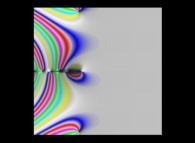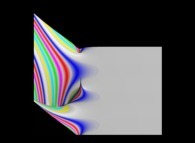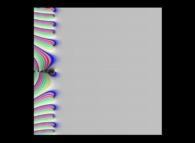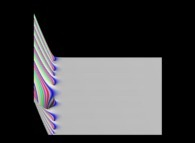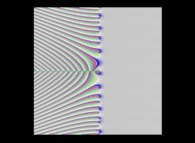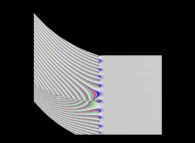Here are some more pictures about the Zeta function:

(CMAP28 WWW site: this page was created on 06/18/1999 and last updated on 02/08/2022 21:04:51 -CET-)

[See all related pictures (including this one) [Voir toutes les images associées (incluant celle-ci)]]

[Please visit the related NumberTheory picture gallery [Visitez la galerie d'images NumberTheory associée]]

[Go back to AVirtualMachineForExploringSpaceTimeAndBeyond [Retour à AVirtualMachineForExploringSpaceTimeAndBeyond]]

[The Y2K Bug [Le bug de l'an 2000]]

[Site Map, Help and Search [Plan du Site, Aide et Recherche]]
[Mail [Courrier]]
[About Pictures and Animations [A Propos des Images et des Animations]]